#Convolution ProductOne of the most common mistake students will commit is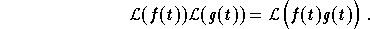Although it is tempting to assume that this is true, one may easily check that it is wrong by taking f(t) = t, and g(t) = t. Therefore, a natural question is: what would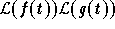be equal to ?
The answer to this question is given by the formula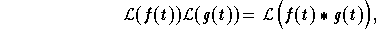where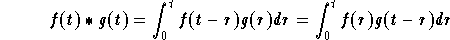,

is called the convolution product of f(t), and g(t). The following are some of the basic properties of the convolution product:

(1)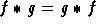;
(2)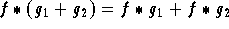;
(3)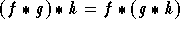;
(4)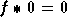;
(5)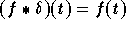.

Example: Find the solution to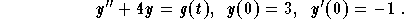Solution: Apply the Laplace transform to get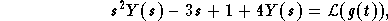where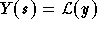. Hence,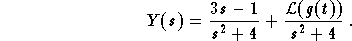We then rewrite Y(s) to get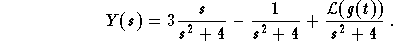Therefore, we have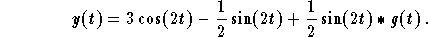[Differential Equations] [First Order D.E.] [Second Order D.E.]
[Geometry] [Algebra] [Trigonometry ]
[Calculus] [Complex Variables] [Matrix Algebra]S.O.S MATHematics home page

Do you need more help? Please post your question on our S.O.S. Mathematics CyberBoard.Author: Mohamed Amine Khamsi